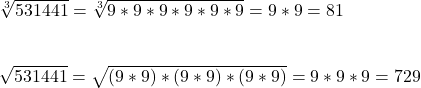Question

Which of the following numbers is both a perfect square and a perfect cube?
i. 12 544
ii. 531 441
iii. 456 235
This is really stumping me up

1.bonexptip

ii. 531441

Step-by-step explanation:

Find the prime factorization of each number.

For a number to be a perfect square, it must have an even number of each factor.

For a number to be a perfect cube, it must have a multiple of three of each factor.

i. 12544 = 2^8 * 7^2

There 8 factors of 2 and 2 factors of 7. The numbers of factors of both 2 and 7 are even, so it’s a perfect square, but neither 8 nor 2 is a multiple of 3, so it is not a perfect cube.

ii. 531441 = 3^12

12 is both even and a multiple of 3, so 531441 is a perfect square and a perfect cube.

iii. 456235 = 5 * 13 * 7019

No factor is a multiple of 2 or 3, so it is not a perfect square of perfect cube.

2.